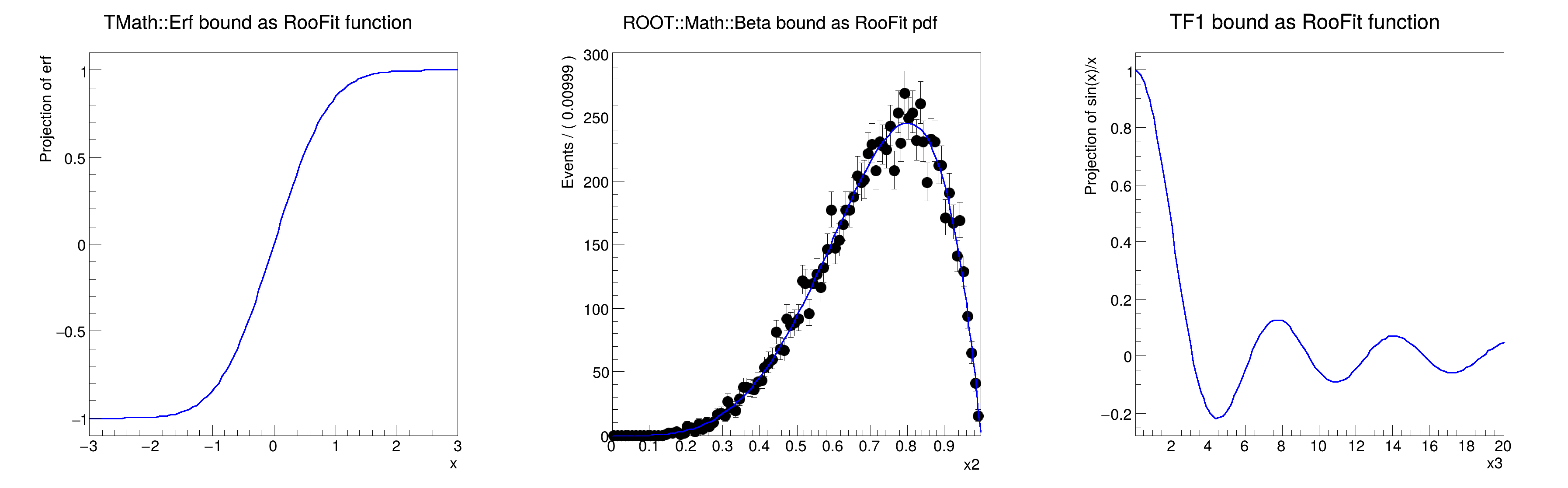ROOT   Reference Guide
Searching...
No Matches
rf105_funcbinding.C File Reference

## Detailed DescriptionBasic functionality: binding ROOT math functions as RooFit functions and pdfs#include "RooRealVar.h"
#include "RooDataSet.h"
#include "RooGaussian.h"
#include "TCanvas.h"
#include "TAxis.h"
#include "RooPlot.h"
#include "TMath.h"
#include "TF1.h"
#include "Math/DistFunc.h"
#include "RooTFnBinding.h"
using namespace RooFit;
{
// B i n d T M a t h : : E r f C f u n c t i o n
// ---------------------------------------------------
// Bind one-dimensional TMath::Erf function as RooAbsReal function
RooRealVar x("x", "x", -3, 3);
RooAbsReal *errorFunc = bindFunction("erf", TMath::Erf, x);
// Print erf definition
errorFunc->Print();
// Plot erf on frame
RooPlot *frame1 = x.frame(Title("TMath::Erf bound as RooFit function"));
errorFunc->plotOn(frame1);
// B i n d R O O T : : M a t h : : b e t a _ p d f C f u n c t i o n
// -----------------------------------------------------------------------
// Bind pdf ROOT::Math::Beta with three variables as RooAbsPdf function
RooRealVar x2("x2", "x2", 0, 0.999);
RooRealVar a("a", "a", 5, 0, 10);
RooRealVar b("b", "b", 2, 0, 10);
RooAbsPdf *beta = bindPdf("beta", ROOT::Math::beta_pdf, x2, a, b);
// Perf beta definition
beta->Print();
// Generate some events and fit
std::unique_ptr<RooDataSet> data{beta->generate(x2, 10000)};
beta->fitTo(*data, PrintLevel(-1));
// Plot data and pdf on frame
RooPlot *frame2 = x2.frame(Title("ROOT::Math::Beta bound as RooFit pdf"));
data->plotOn(frame2);
beta->plotOn(frame2);
// B i n d R O O T T F 1 a s R o o F i t f u n c t i o n
// ---------------------------------------------------------------
// Create a ROOT TF1 function
TF1 *fa1 = new TF1("fa1", "sin(x)/x", 0, 10);
// Create an observable
RooRealVar x3("x3", "x3", 0.01, 20);
// Create binding of TF1 object to above observable
RooAbsReal *rfa1 = bindFunction(fa1, x3);
// Print rfa1 definition
rfa1->Print();
// Make plot frame in observable, plot TF1 binding function
RooPlot *frame3 = x3.frame(Title("TF1 bound as RooFit function"));
rfa1->plotOn(frame3);
TCanvas *c = new TCanvas("rf105_funcbinding", "rf105_funcbinding", 1200, 400);
c->Divide(3);
c->cd(1);
frame1->GetYaxis()->SetTitleOffset(1.6);
frame1->Draw();
c->cd(2);
frame2->GetYaxis()->SetTitleOffset(1.6);
frame2->Draw();
c->cd(3);
frame3->GetYaxis()->SetTitleOffset(1.6);
frame3->Draw();
}
#define b(i)
Definition RSha256.hxx:100
#define c(i)
Definition RSha256.hxx:101
#define a(i)
Definition RSha256.hxx:99
Option_t Option_t TPoint TPoint const char GetTextMagnitude GetFillStyle GetLineColor GetLineWidth GetMarkerStyle GetTextAlign GetTextColor GetTextSize void data
Option_t Option_t TPoint TPoint const char x2
void Print(Option_t *options=nullptr) const override
Print the object to the defaultPrintStream().
Definition RooAbsArg.h:323
RooAbsReal is the common abstract base class for objects that represent a real value and implements f...
Definition RooAbsReal.h:59
virtual RooPlot * plotOn(RooPlot *frame, const RooCmdArg &arg1={}, const RooCmdArg &arg2={}, const RooCmdArg &arg3={}, const RooCmdArg &arg4={}, const RooCmdArg &arg5={}, const RooCmdArg &arg6={}, const RooCmdArg &arg7={}, const RooCmdArg &arg8={}, const RooCmdArg &arg9={}, const RooCmdArg &arg10={}) const
Plot (project) PDF on specified frame.
A RooPlot is a plot frame and a container for graphics objects within that frame.
Definition RooPlot.h:43
static RooPlot * frame(const RooAbsRealLValue &var, double xmin, double xmax, Int_t nBins)
Create a new frame for a given variable in x.
Definition RooPlot.cxx:239
TAxis * GetYaxis() const
Definition RooPlot.cxx:1279
void Draw(Option_t *options=nullptr) override
Draw this plot and all of the elements it contains.
Definition RooPlot.cxx:652
RooRealVar represents a variable that can be changed from the outside.
Definition RooRealVar.h:37
virtual void SetTitleOffset(Float_t offset=1)
Set distance between the axis and the axis title.
Definition TAttAxis.cxx:298
The Canvas class.
Definition TCanvas.h:23
1-Dim function class
Definition TF1.h:213
double beta_pdf(double x, double a, double b)
Probability density function of the beta distribution.
Double_t x[n]
Definition legend1.C:17
The namespace RooFit contains mostly switches that change the behaviour of functions of PDFs (or othe...
Double_t Erf(Double_t x)
Computation of the error function erf(x).
Definition TMath.cxx:190
RooCFunction1Binding<double,double>::erf[ function=TMath::Erf x=x ] = 0
RooCFunction3PdfBinding<double,double,double,double>::beta[ function=(0x7f67fa106e70) x=x2 y=a z=b ] = 0.934689
[#1] INFO:NumericIntegration -- RooRealIntegral::init(beta_Int[x2]) using numeric integrator RooRombergIntegrator to calculate Int(x2)
[#1] INFO:NumericIntegration -- RooRealIntegral::init(beta_Int[x2]) using numeric integrator RooRombergIntegrator to calculate Int(x2)
[#1] INFO:NumericIntegration -- RooRealIntegral::init(beta_Int[x2]) using numeric integrator RooRombergIntegrator to calculate Int(x2)
[#1] INFO:Minimization -- RooAbsMinimizerFcn::setOptimizeConst: activating const optimization
[#1] INFO:Minimization -- RooAbsMinimizerFcn::setOptimizeConst: deactivating const optimization
[#1] INFO:NumericIntegration -- RooRealIntegral::init(beta_Int[x2]) using numeric integrator RooRombergIntegrator to calculate Int(x2)
RooTFnBinding::fa1[ TFn={fa1=sin(x)/x} obs=(x3) ] = -0.0547936
Date
July 2008

Definition in file rf105_funcbinding.C.